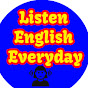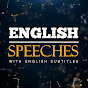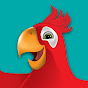# Practice English Speaking&Listening with: Math Antics - Fractions Are Parts

Normal
(0)
Difficulty: 0

If youve been learning math for a while,

And if youve ever had to break a cookie in half to share with a friend,

well then youve used fractions in real life.

Ohwell, thank youMmmmm!

Thats because fractions are used to represent smaller pieces or parts of things.

When youre first learning about fractions, it can help to draw pictures to see how they work.

So lets start by drawing a circle.

This circle represents what we call a whole.

No, not the kind of hole that you could fall into!

More like a whole cookie, or a whole pizza.

Now, if we divide that circle, or whole amount, into four parts,

then we call each of those parts a fraction of the original whole circle.

Because this is Math class and not Art, we cant just keep on drawing pictures.

We need to learn how to write out fractions using the language of math.

Yep, you guessed it! That means using numbers.

Unlike a regular number, to write a fraction you need two numbers;

one number on top, one number on bottom, and a line between them.

Ill explain exactly what the line is for later in the video,

but for now, lets figure out what the two numbers mean.

Remember, fractions are used to represent parts of something,

and the number on the bottom tells us how many parts that something is divided into.

The number on top tells us how many of those parts we have.

['elevator' music]

For example, lets write a fraction for our drawing here.

I divided the circle into four parts, right?

So the number on the bottom will be4’.

And I still have all four of those parts, so the number on top will also be4’.

I have4out of4parts. That means I still have a whole circle.

But what if someone came by and took away one part from the circle.

Wellthe circle is still divided into four parts, so the bottom number would still be4’.

But I only have three of those parts left, so that means the top number will change to3’.

So I have3over4’, or three-fourths of the circle.

make sense so far?

Good! Lets try another example.

Lets say that I divide a rectangle up into eight parts, and I give you three of those parts.

Since the total number of parts is eight, the bottom number will be8’,

and since you have three of those parts, the top number will be3’.

So the fraction Im giving you is… ‘3over8’, or three-eighths of the rectangle.

No, no, nono need to thank me.

Ohand its important to remember that for fractions to work right,

the parts that you divide the whole up into have to be equal.

We cant take likewelltake a candy bar and say,

well Im gonna divide it into two parts. So this is your half, and here's my half”.

Lets look at a few more examples

so you can really see the pattern of how fractions can represent parts of objects.

This rectangle is divided up into three equal parts, and two of them are shaded red.

So2over3’, or two-thirds of the rectangle is shaded red.

This circle is divided into twelve equal parts, and seven of those parts are shaded green.

So, ‘7over12’, or seven-twelfths of the circle is shaded green.

This hexagon is divided into six equal parts, and five of those parts are shaded blue.

So5over6’, or five-sixths of the hexagon is shaded blue.

Alright, so you can see how fractions can be used to represent parts of objects like circles and rectangles,

but fractions can be used for more than that.

They can be used to represent parts ofwell, anything!

Likelike pets for example.

Lets say you have seven pets;

four dogs,

two cats,

and a big, fat, hamster.

That means that4over7’, or four-sevenths of your pets are dogs,

and2over7’, or two-sevenths of your pets are cats,

and1over7’, or one-sevenths of your pets are hamsters.

It also means that your house probably smells like a pet store. [laughter]

Okayso fractions can be used to represent anything from parts of a circle to kitty-cats.

But did you know that fractions can also be used represent things you cant even see?

Well, like a test score for example

Lets say you take a math test and theres 20 questions on the test,

and you get 17 of those questions right.

That means that you got17over20’ , or seventeen-twentieths of the questions right.

Alrightso thats the basic idea of how fractions can be used to represent parts of things,

but theres a lot more to fractions than that.

In the next section, were going to be looking at some of the other things we can do with fractions.

But before that, lets review

Fractions are used to represent parts of a whole.

Fractions are written in the form of a top number over a bottom number with a line between them.

The bottom number represents how many parts the whole is divided into.

And the top number represents how many of those parts you have.

And finallythe parts that a whole is divided up into must be equal for fractions to work right.

To really understand how fractions are used to represent parts of things,

be sure to do the exercises, and Ill see you in section two.

•Learn English With TV Series
•Listen English Everyday
•Daily English Conversation
•English Speeches
•Langfocus
•Eko Languages
•The English Coach
•linguamarina